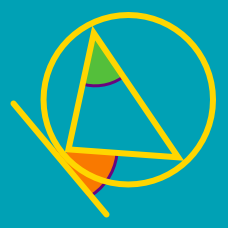Geometry

# Circles - Intersecting Chords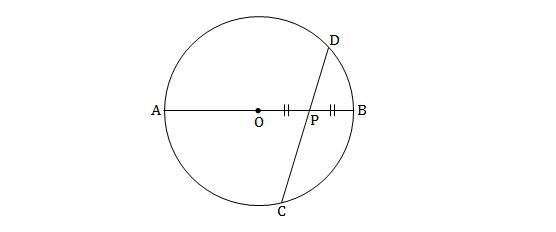Let $r$ be the radius of the above circle centered at $O.$ If $\begin{array}{c}\displaystyle \lvert{\overline{PD}}\rvert=10, & \lvert{\overline{PC}}\rvert=15\end{array}$ and $P$ is the midpoint of $\overline{OB},$ what is $r^2?$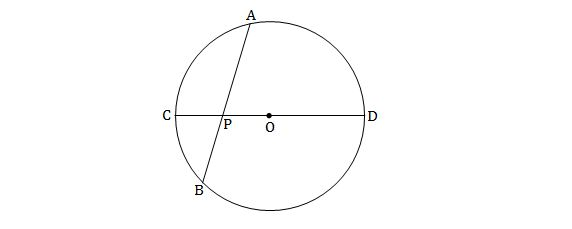The lengths of some line segments in the figure above are $\begin{array}{c}\displaystyle \lvert{\overline{AP}}\rvert=9, & \lvert{\overline{BP}}\rvert=6, & \lvert{\overline{OD}}\rvert=9.\end{array}$ If $\lvert{\overline{OP}}\rvert=x,$ then what is the value of $x^2 ?$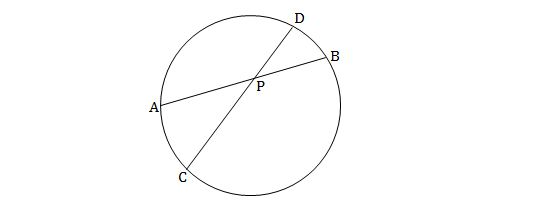The lengths of some line segments in the figure above are $\begin{array}{c}\displaystyle \lvert{\overline{AP}}\rvert=13, & \lvert{\overline{CP}}\rvert=17, & \lvert{\overline{DP}}\rvert=8.\end{array}$ Then what is $\lvert{\overline{BP}}\rvert ?$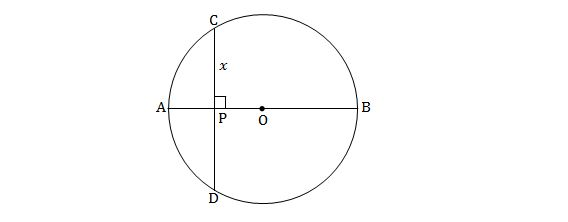If the lengths of some line segments in the figure above are $\begin{array}{c}\displaystyle \lvert{\overline{AP}}\rvert=6, & \lvert{\overline{OB}}\rvert=8, & \lvert{\overline{CP}}\rvert=x,\end{array}$ then what is the value of $x^2 ?$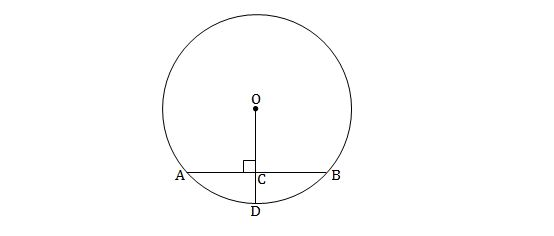In the above circle centered at $O,$ let $\lvert{\overline{OD}}\rvert=16,$ $\lvert{\overline{CD}}\rvert=8$ and $\overline{OD} \perp \overline{AB}.$ Then what is the value of $\lvert{\overline{CA}}\rvert^2 ?$

×